# Au Revoir Backprop! Bonjour Optical Transfer Learning!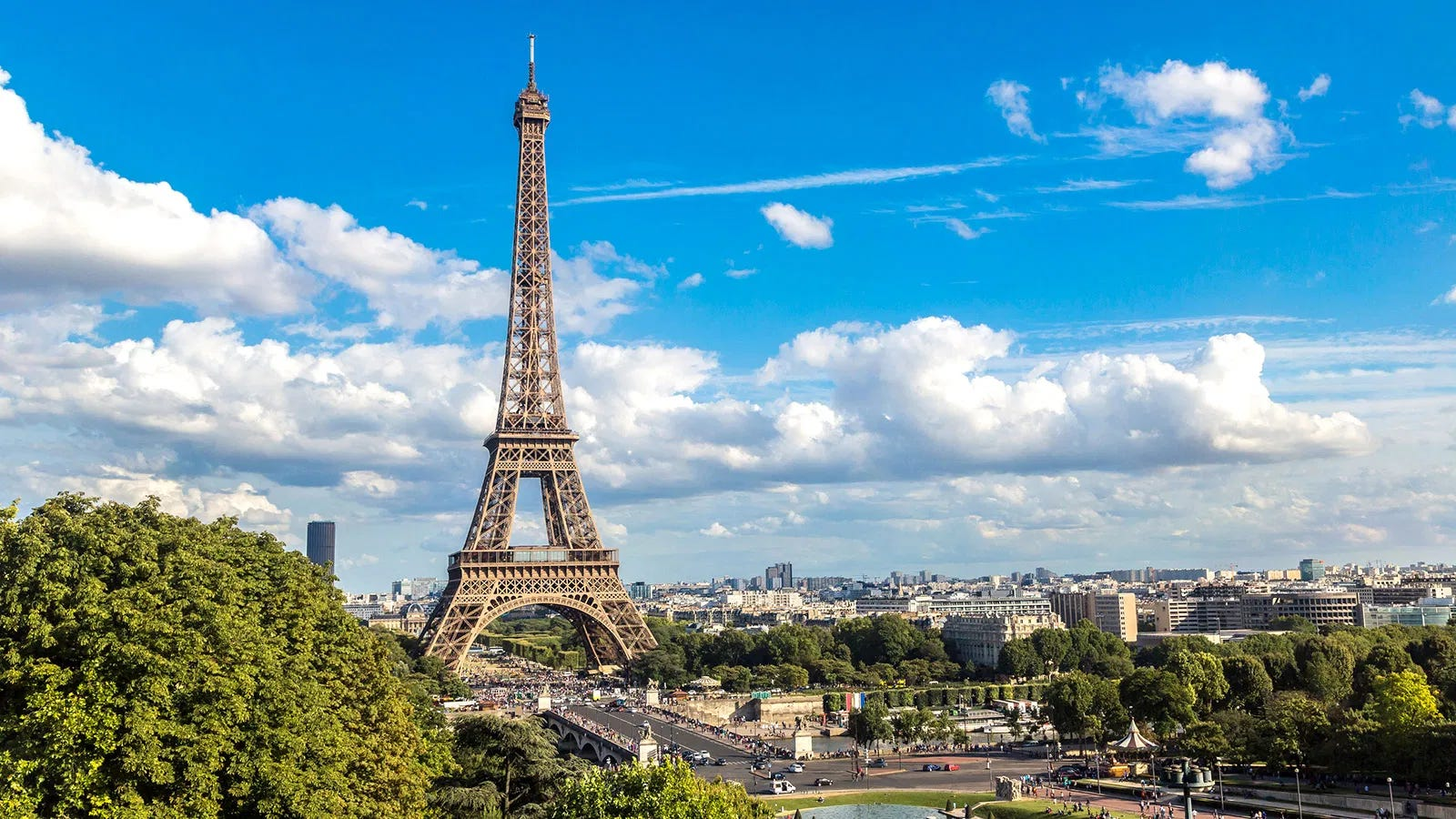A view of Paris

As humans, whenever we learn something new, we build upon our past experience and knowledge. Transfer learning is the same powerful idea but applied to deep learning.

What is Transfer Learning?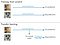Figure 1: A schematic representation of transfer learning on the Animals10 datasets. Picture credits to A. Chatelain.

Transfer learning is widely used in Deep Learning. It aims to pass part of the knowledge gained by one model in solving one task over to another model. It is useful when available data is limited, or when time is a big constraint in training a model. In this blog post, we will focus on an application in the field of image classification. Interesting results have also been reached in other domains, such as natural language processing  and video action recognition .

Let’s start with an example. Suppose that we have to create two models: one to discriminate between breeds of dogs and one between breeds of cats. We could train the two models from scratch separately on their respective datasets. However, there is a smarter and faster approach: train our first model on the cats' dataset, then use it to initialize the second model and train it on the dogs' images. The two datasets share some features: for example, cats and dogs both have four legs and one tail. Since our network has learned the definition of these features from the first dataset, we get a headstart in the training on the second dataset.

Generally, a model is chosen and trained on a dataset that shares some similarities to the target dataset. The common choice is typically the ImageNet dataset . Most of the state-of-the-art Convolutional Neural Networks (CNN) like VGG, ResNets, or DenseNets pre-trained on ImageNet are available by default in ML frameworks such as Pytorch.

A different approach to transfer learning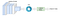Figure 2: Schematic representation of the Transfer Learning pipeline with the OPU.

How do we use our OPU to perform transfer learning? First of all, we remove the linear layers at the end of the network to extract the convolutional features associated with the input images. The feature matrix will have size (), where is the number of image samples, and the number of convolutional features

The OPU pipeline to perform transfer learning on an image dataset is the following one:

• We pass the images through a CNN to extract the convolutional features.
• Since the input to the OPU needs to be binary, we encode the convolutional features according to their sign: +1 if positive, 0 if negative. Even though this might seem very lossy, it works surprisingly well.
• The matrix of convolutional features of size is passed through the OPU to compute the matrix of random features . Choosing k > m projects the data into a higher-dimensional space where it might be linearly separable. On the other hand, by picking k < m we reduce the overall size of the model and accelerate the training at the expense of the test accuracy.
• We fit a linear classifier like Logistic regression or Ridge on the random features of the training set.

The steps for the inference phase are the same, with the linear model fitted at training time giving the class predictions for each sample.

We compare this approach with the standard backpropagation algorithm in a variety of different settings. In particular, we show that:

• The OPU approach can yield comparable accuracy with respect to the standard backpropagation procedure in a smaller training time since it does not require gradient computation or multiple passes over the data;
• The OPU requires less time in the choice of the hyperparameters for the simulation. There is no learning rate or momentum to adjust, nor any particular sensitivity to the batch size. The only hyperparameters are the number of projections, the regularization parameter for the Ridge Classifier and the index of the last layer in the network;
• We can train in lower precision arithmetics such as float16 or int8 and still obtain high test accuracy with lower training time and memory consumption since we do not have instability problems associated with gradients.

Using the OPU and DenseNet-169 to perform animal classification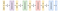Figure 3: Example structure of a DenseNet architecture.

We use theDenseNet169 model  pre-trained on ImageNet throughout this blog post. We picked this model because of its depth: with the OPU, we can remove parts of the architecture and still achieve competitive results in terms of accuracy and training time. Figure 3 shows the model architecture.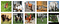Figure 4: Samples from each class of the Animals10 dataset.

We train the model on the Animal-10 dataset available at this link.

It consists of 24,209 images belonging to 10 different classes, that we divided into a random split with an 80:20 ratio between train and test images (19,363 train images versus 4,846 test images). A preview of the images is in Figure 4.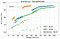Figure 5: Test accuracy [%] as a function of the model size for the Animals10 dataset trained on the DenseNet169 model. The green points correspond to the accuracy of a model trained with backpropagation. The blue and orange points refer to the same model trained with the OPU on float32 and float16 features respectively.

Figure 5 shows the test accuracy as a function of the model size. The model trained with the OPU is more accurate than the backpropagation baseline. This becomes more evident as more layers are removed from the architecture. Further training and hyperparameter tuning with backpropagation might increase the final test accuracy, but our alternative approach has the advantage to work out of the box.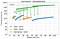Figure 6: Training time [s] as a function of the model size for the Animals10 dataset trained on the DenseNet169 model. The green points correspond to the accuracy of a model trained with backpropagation. The blue and orange points refer to the same model trained with the OPU on float32 and float16 features respectively. The dips are due to the fact that the model with the highest accuracy was reached in a lower amount of training epochs than the others.

Furthermore, we can train the model five to six times faster depending on the number of removed layers as shown in Figure 6. We could achieve further speedup by lowering the number of random projections. This would reduce the time it takes to fit the Ridge Classifier at the expense of the test accuracy.

Additionally, we trained the model on the convolutional features of the dataset fully extracted in float16. Since our method is gradient-free, we have no risk of underflow or overflow in the gradients. This means that we can reduce the model size by half and accelerate the training without virtually any cost.

We do not observe a large gain in training time between float32 and float16, but this is due to the GPU model used (NVIDIA P100). A different model like an NVIDIA V100 or RTX 2080 with better half-precision capabilities would be almost twice as fast in extracting the convolutional features.

Low precision training: a low-hanging fruit

We can further reduce the training time and model size by performing the training in int8. At the time of the experiments, Pytorch did not support int8 computations, so we decided to use the TensorRT library developed by NVIDIA available here. An overview of how int8 quantization is performed with TensorRT is described here.

The training pipeline does not change: the only difference is that the TensorRT library converts all the weights and activations of the network in int8. This allows us, in turn, to perform computations directly on 8-bit integers, which are often two to four times faster than in float32 and reduce the memory bandwidth by a factor four. There is a caveat: the GPU model must support int8 computations. This is not the case for the NVIDIA P100 GPU, so we switched to the RTX 2080 for that. We also run the same simulation in float32 and float16 using TensorRT on the RTX to provide a fair comparison.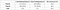Table 1: Accuracy [%] and Training time [s] on the DenseNet169 trained in different numerical precisions with the OPU pipeline. The model was cut to the 12th DenseLayer in the last DenseBlock. In the parenthesis the results obtained with Pytorch when applicable.

We picked the network configuration that performs on par with the backpropagation baseline in terms of accuracy. Table 1 shows a comparison of the accuracy of the same model at different numerical precisions.

We lost less than 1% in accuracy, but reduced memory consumption by a factor 4 with respect to the float32 model; pretty satisfying!

Even though the time to compute the convolutions is halved, the total training time does not substantially decrease, because it is dominated by the solution of the Ridge problem.

The next step could be to implement the Ridge classifier on GPU to reap the benefits of the lower precision arithmetic and further accelerate the training.

Bibliography

 Baevski, Alexei, et al. “Cloze-driven pretraining of self-attention networks.” (2019).

 Carreira, Joao, and Andrew Zisserman. “Quo vadis, action recognition? a new model and the kinetics dataset.” . 2017

 Olga Russakovsky*, Jia Deng*, Hao Su, Jonathan Krause, Sanjeev Satheesh, Sean Ma, Zhiheng Huang, Andrej Karpathy, Aditya Khosla, Michael Bernstein, Alexander C. Berg, and Li Fei-Fei. (* = equal contribution) ImageNet Large Scale Visual Recognition Challenge. , 2015.

 Huang, G., et al. “Densely Connected Convolutional Networks. arXiv 2017.”

We are a technology company developing Optical Computing for Machine Learning. Our tech harvests Computation from Nature, We are at lighton.ai

## More from LightOn

We are a technology company developing Optical Computing for Machine Learning. Our tech harvests Computation from Nature, We are at lighton.ai